Examples from NCERT Book

Class 10
Chapter 12 Class 10 - Electricity

## (b) the total current flowing in the circuit.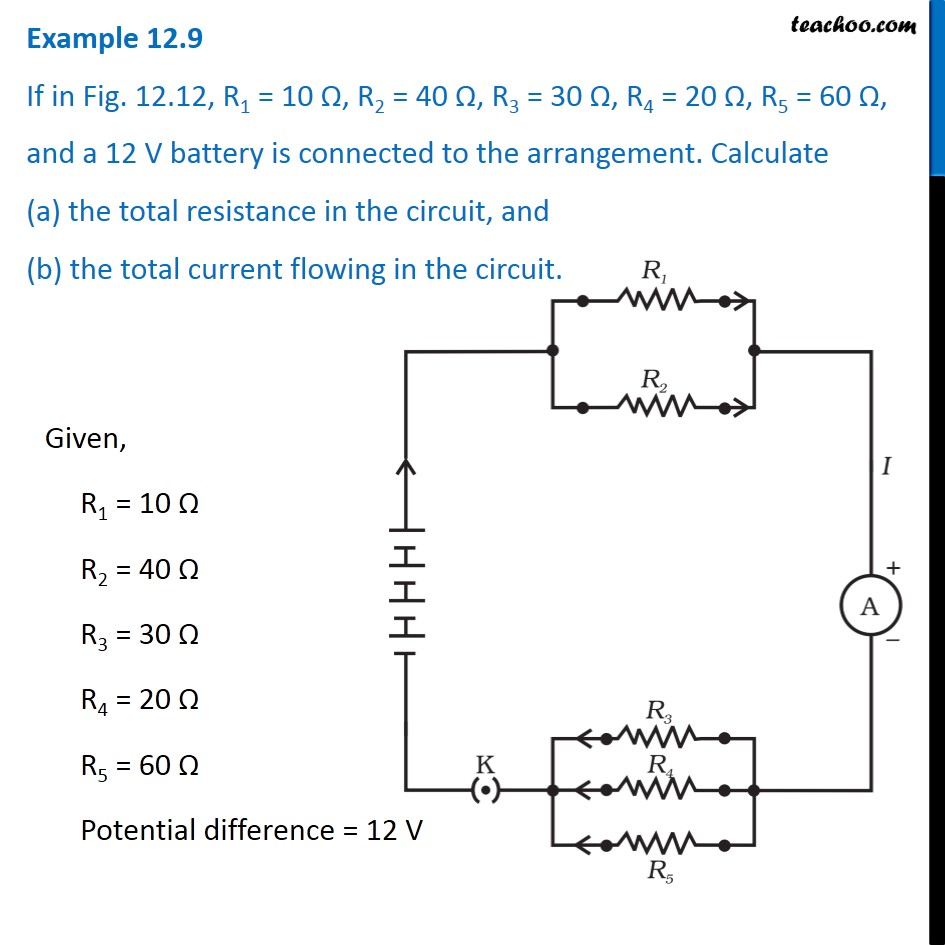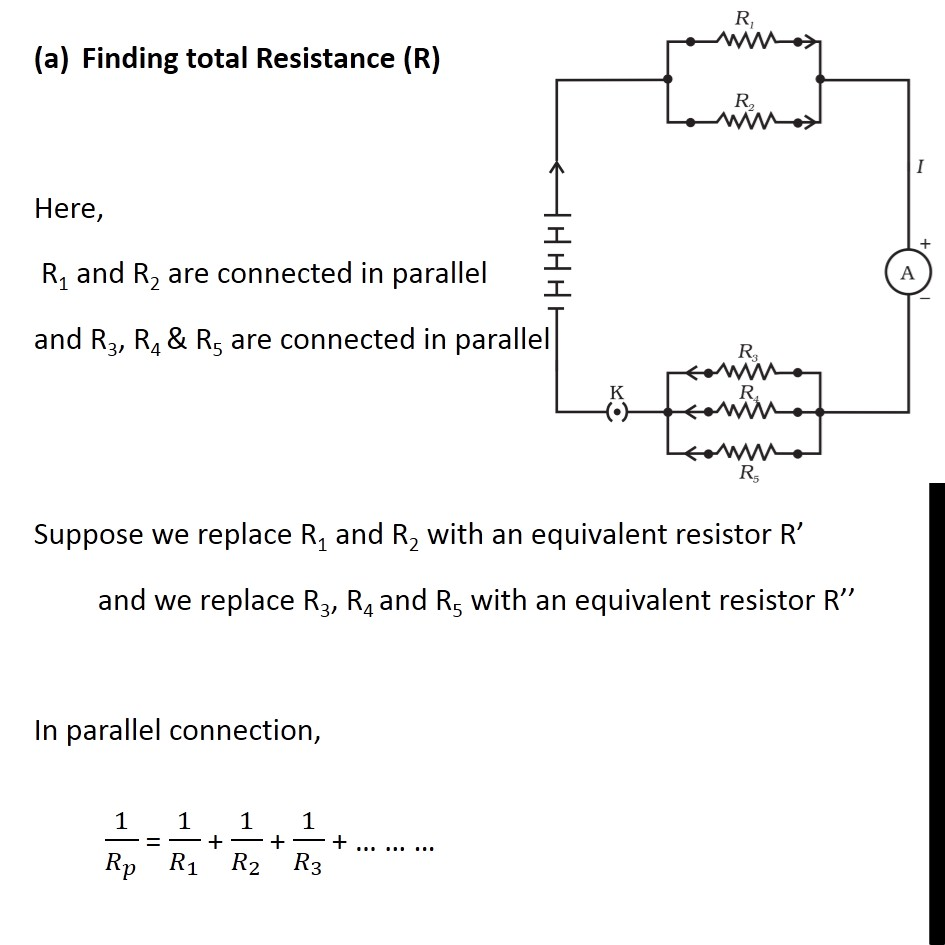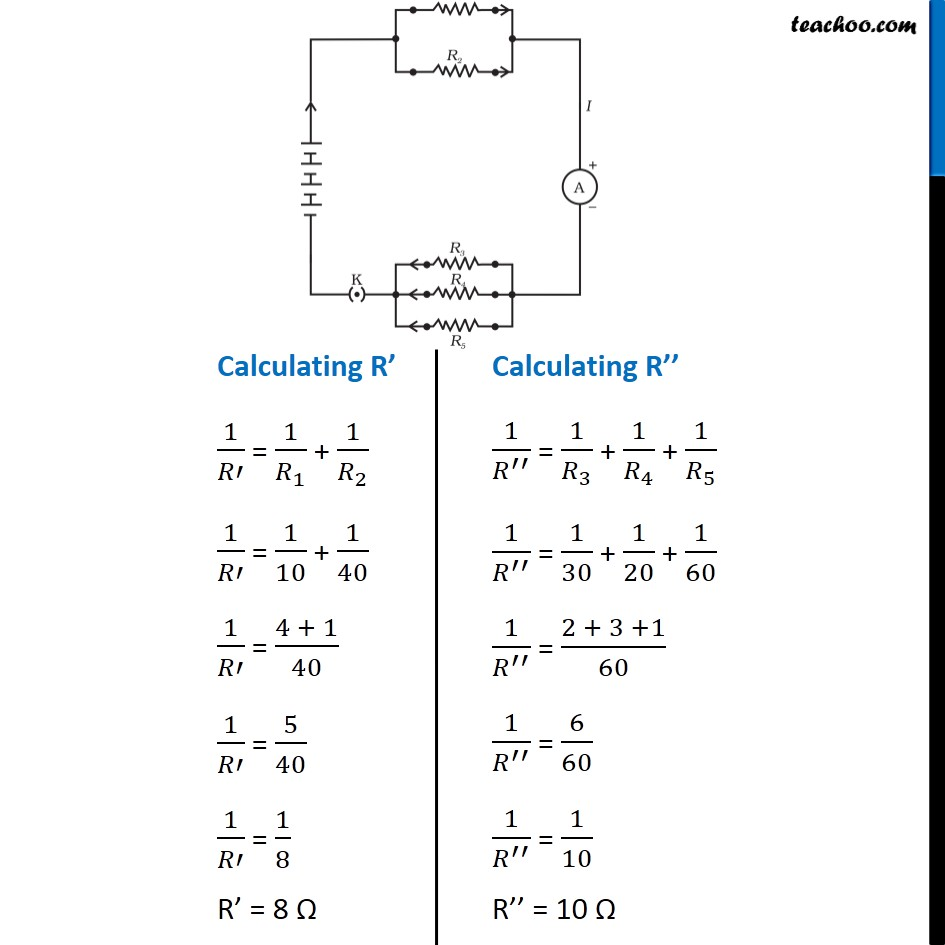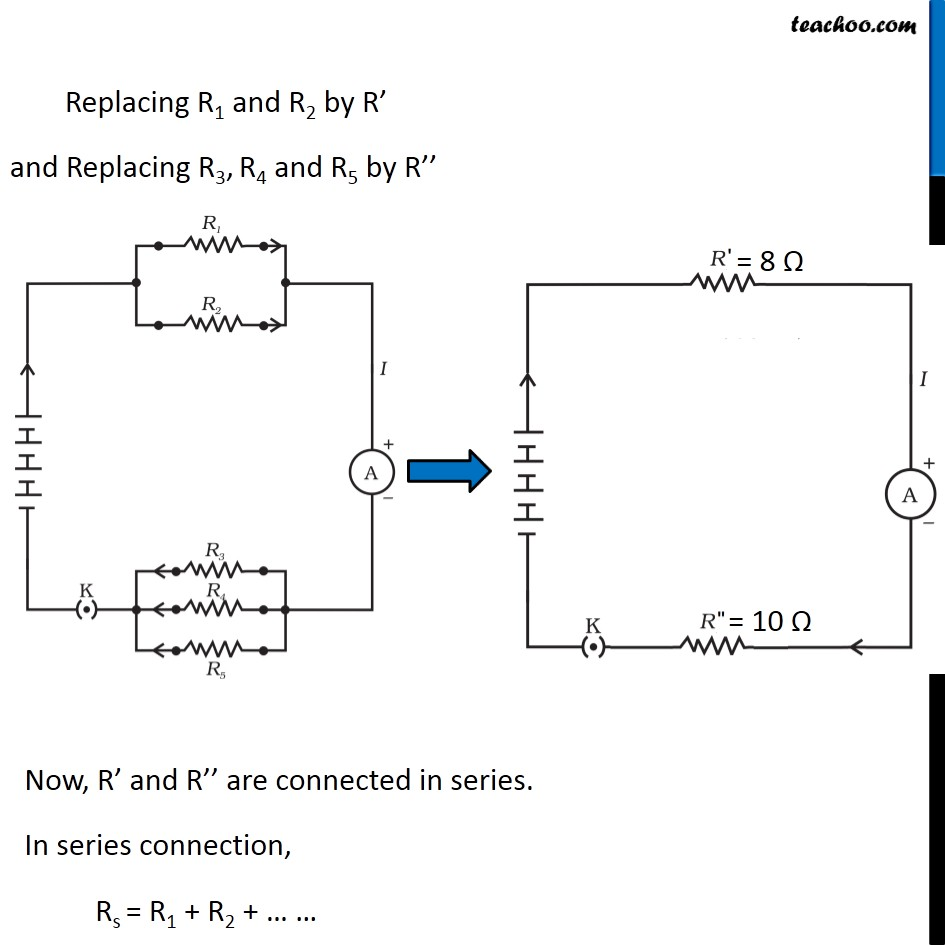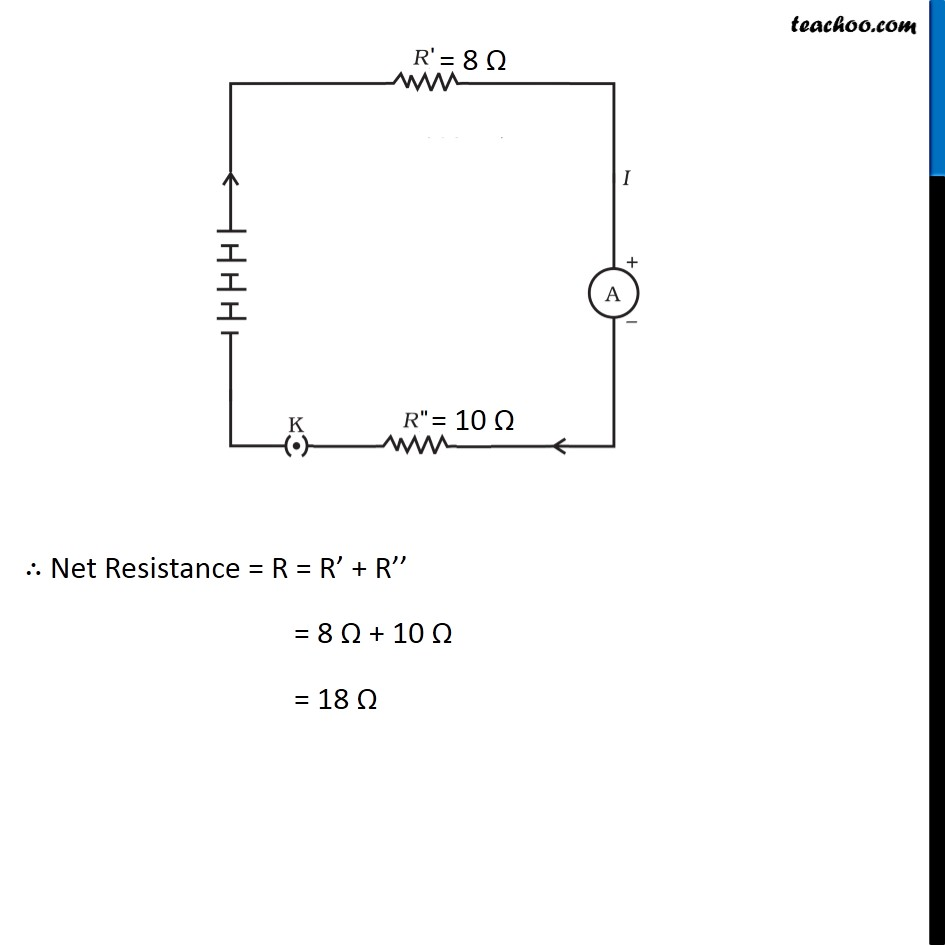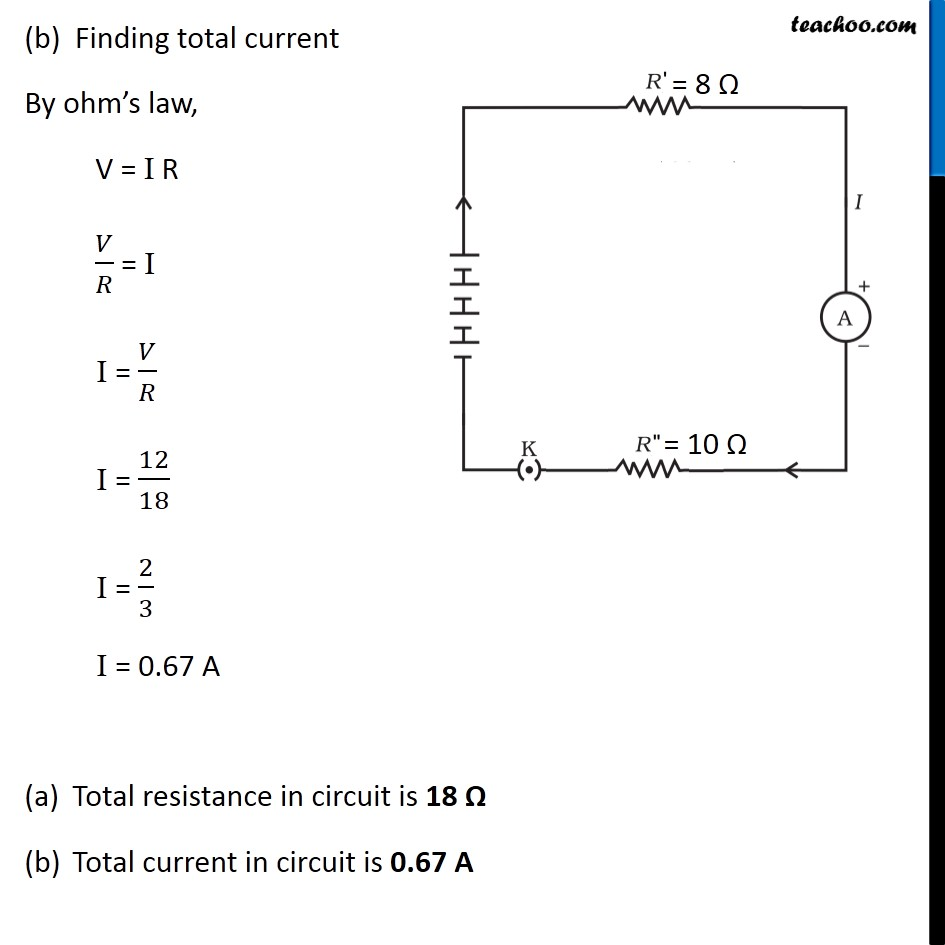Learn in your speed, with individual attention - Teachoo Maths 1-on-1 Class

### Transcript

Example 12.9 If in Fig. 12.12, R1 = 10 Ω, R2 = 40 Ω, R3 = 30 Ω, R4 = 20 Ω, R5 = 60 Ω, and a 12 V battery is connected to the arrangement. Calculate (a) the total resistance in the circuit, and (b) the total current flowing in the circuit. Given, R1 = 10 Ω R2 = 40 Ω R3 = 30 Ω R4 = 20 Ω R5 = 60 Ω Potential difference = 12 V Finding total Resistance (R) Here, R1 and R2 are connected in parallel and R3, R4 & R5 are connected in parallel Suppose we replace R1 and R2 with an equivalent resistor R’ and we replace R3, R4 and R5 with an equivalent resistor R’’ In parallel connection, 1/𝑅_𝑝 = 1/𝑅_1 + 1/𝑅_2 + 1/𝑅_3 + … … … Calculating R’ 1/𝑅′ = 1/𝑅_1 + 1/𝑅_2 1/𝑅′ = 1/10 + 1/40 1/𝑅′ = (4 + 1)/40 1/𝑅′ = 5/40 1/𝑅′ = 1/8 R’ = 8 Ω Calculating R’’ 1/𝑅^′′ = 1/𝑅_3 + 1/𝑅_4 + 1/𝑅_5 1/𝑅^′′ = 1/30 + 1/20 + 1/60 1/𝑅^′′ = (2 + 3 +1)/60 1/𝑅^′′ = 6/60 1/𝑅^′′ = 1/10 R’’ = 10 Ω Replacing R1 and R2 by R’ and Replacing R3, R4 and R5 by R’’ Now, R’ and R’’ are connected in series. In series connection, Rs = R1 + R2 + … … ∴ Net Resistance = R = R’ + R’’ = 8 Ω + 10 Ω = 18 Ω (b) Finding total current By ohm’s law, V = I R 𝑉/𝑅 = I I = 𝑉/𝑅 I = 12/18 I = 2/3 I = 0.67 A Total resistance in circuit is 18 Ω Total current in circuit is 0.67 A